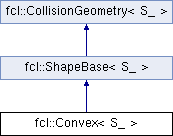FCL  0.6.0 Flexible Collision Library
fcl::Convex< S_ > Class Template Reference

Convex polytope. More...

`#include <convex.h>`

Inheritance diagram for fcl::Convex< S_ >:struct  Edge

## Public Types

using S = S_Public Types inherited from fcl::ShapeBase< S_ >
using S = S_

## Public Member Functions

Convex (Vector3< S > *plane_normals, S *plane_dis, int num_planes, Vector3< S > *points, int num_points, int *polygons)
Constructing a convex, providing normal and offset of each polytype surface, and the points and shape topology information.

Convex (const Convex &other)
Copy constructor.

void computeLocalAABB () override
Compute AABB<S>

NODE_TYPE getNodeType () const override
Get node type: a conex polytope.

Matrix3< S > computeMomentofInertia () const override
based on http://number-none.com/blow/inertia/bb_inertia.doc

Vector3< S > computeCOM () const override
compute center of mass

computeVolume () const override
compute the volume

std::vector< Vector3< S > > getBoundVertices (const Transform3< S > &tf) const
get the vertices of some convex shape which can bound this shape in a specific configurationPublic Member Functions inherited from fcl::ShapeBase< S_ >
OBJECT_TYPE getObjectType () const
Get object type: a geometric shape.Public Member Functions inherited from fcl::CollisionGeometry< S_ >
void * getUserData () const
get user data in geometry

void setUserData (void *data)
set user data in geometry

bool isOccupied () const
whether the object is completely occupied

bool isFree () const
whether the object is completely free

bool isUncertain () const
whether the object has some uncertainty

virtual Matrix3< S_ > computeMomentofInertiaRelatedToCOM () const
compute the inertia matrix, related to the com

## Public Attributes

Vector3< S > * plane_normals

S * plane_dis

int * polygons
An array of indices to the points of each polygon, it should be the number of vertices followed by that amount of indices to "points" in counter clockwise order.

Vector3< S > * points

int num_points

int num_edges

int num_planes

Edgeedges

Vector3< S > center
center of the convex polytope, this is used for collision: center is guaranteed in the internal of the polytope (as it is convex)Public Attributes inherited from fcl::CollisionGeometry< S_ >
Vector3< S_ > aabb_center
AABB center in local coordinate.

AABB< S_ > aabb_local
AABB in local coordinate, used for tight AABB when only translation transform.

void * user_data
pointer to user defined data specific to this object

S_ cost_density
collision cost for unit volume

S_ threshold_occupied
threshold for occupied ( >= is occupied)

S_ threshold_free

## Protected Member Functions

void fillEdges ()
Get edge information.

## Detailed Description

### template<typename S_> class fcl::Convex< S_ >

Convex polytope.

The documentation for this class was generated from the following files:
• /home/js/dev/fcl/master/include/fcl/geometry/shape/convex.h
• /home/js/dev/fcl/master/include/fcl/geometry/shape/convex-inl.h